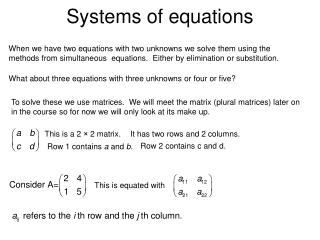# Systems of equations - PowerPoint PPT PresentationDownload PresentationSystems of equations

Systems of equationsDownload Presentation## Systems of equations

- - - - - - - - - - - - - - - - - - - - - - - - - - - E N D - - - - - - - - - - - - - - - - - - - - - - - - - - -
##### Presentation Transcript

1. Systems of equations When we have two equations with two unknowns we solve them using the methods from simultaneous equations. Either by elimination or substitution. What about three equations with three unknowns or four or five? To solve these we use matrices. We will meet the matrix (plural matrices) later on in the course so for now we will only look at its make up. This is a 2 × 2 matrix. It has two rows and 2 columns. Row 2 contains c and d. Row 1 contains a and b. This is equated with

2. R1÷ 2 2R2-R1 We can write a system of equations using the augmented matrix. Written in the form of an augmented matrix we get We then perform Elementary Row Operations (ERO’s) to solve the system. This does look at little complicated but it is the method we use for larger systems.

3. For 3 x 3 systems and larger we use the following strategy. We use ERO’s to reduce this matrix to We want zero’s in the lower half under the leading diagonal. This is often referred to as upper triangular form. We can then solve the system by using substitution.

4. R1 R2-4R1 R3-R1 R1 -R2 R3-R2 Substituting this into row 2, Substituting this into row 1, Hence the solution is x = 1, y = -1, z = 2.

5. This technique of solving a system of equations by expressing the system in augmented matrix form, reducing it to upper triangular form and then performing back substitution is called Gaussian Elimination. It is named after the famous German mathematician Carl Friedrich Gauss. 1777 – 1855 who devised it. Page 127 Exercise 4A Questions 1 and 2. TJ Exercise 1.

6. Redundancy and Inconsistency R1 R2 R3-3R2 R1 R2-R1 R3-4R1 The row of zeros tells us that this equation is redundant, so that there is not a unique solution to the system. There is in fact an infinite number of solutions. Given any z, then from row 2, Sub. This into row 1 Which simplifies to

7. Hence for any value z, A particular solution can be found by assigning a value to z.

8. R1 R2 R3-R2 R1 R2-2R1 R3-3R1 Row 3 suggests that 0 = 1, which tells us that the system of equations is in fact inconsistent and that there are no solutions. Page 130 exercise 5 Questions 1(a) to (c) and 2.

9. Ill- conditioning Consider the following story. Two bags of mixed screws are weighed to the nearest gram. The bags are then sorted out and it is discovered that the situation can be modelled by the system of equations: 19 of one type + 18 of the other weighs 55g 20 of one type + 19 of the other weighs 58g Where x and y are the weights of one of each type of screw, measured in grams. Solving this system leads to the conclusion that type x weigh 1g each and type y weigh 2g each. However, the 55g measurement represents 55 ± 0.5g and the 58g similarly represents 58 ± 0.5g, giving percentage errors of around 1%.

10. 57.5 58 58.5 54.5 55 55.5 (-17.5, 21.5) (-8.5, 12) (0.5, 2.5) (-8, 11.5) (1, 2) (10, -7.5) (1.5, 1.5) (10.5, -8) (19.5, -17.5) Let us look at the different weights. 1850% error 975% error

11. 1850% error 975% error The actual result is being swamped by the error. When, like this, a small change in any of the values in a system of equations leads to a disproportionate change in the solutions then the equations are said to be ill conditioned. When this occurs we have no confidence in the results obtained from such a system. Similar effects occur in larger systems of equations.

12. At the very least we have Solving gives x = 1, y = 2, z = 3. Exploring only the effect of fuzziness of 44: Altering equation 1 to 11x + 12y + 3z = 43.5, gives x = -7.5, y = 11, z = -2 Altering equation 1 to 11x + 12y + 3z = 44.5, gives x = 9.5, y = -7, z = 8 Page 137 Question 2. TJ Exercise 2 Do the Review on page 138